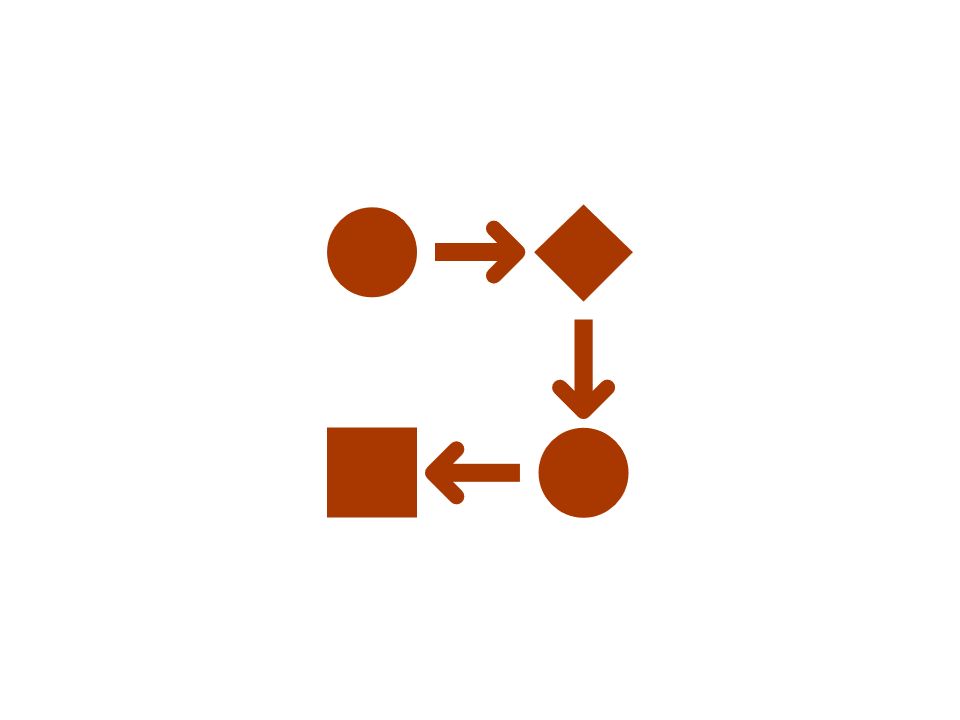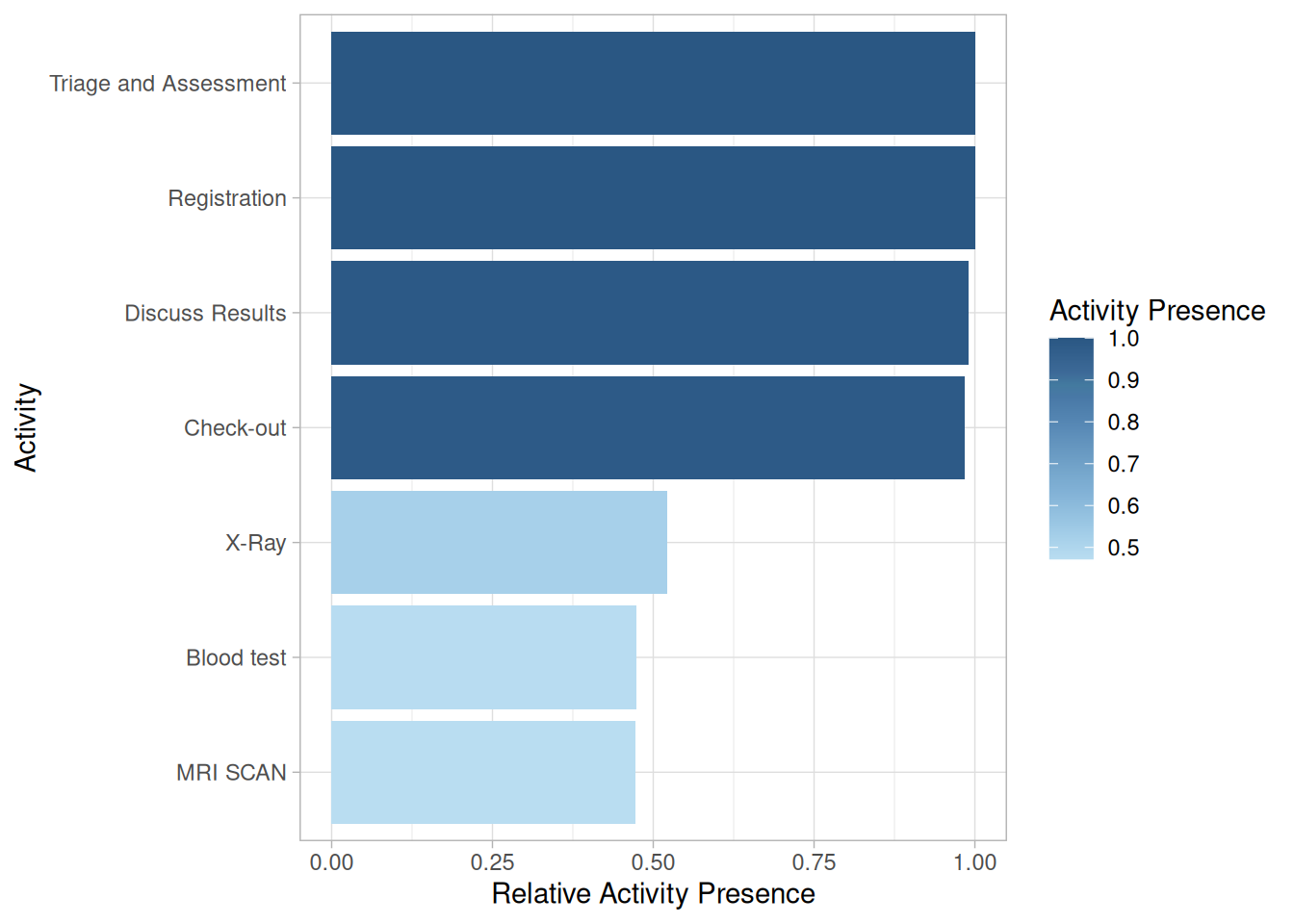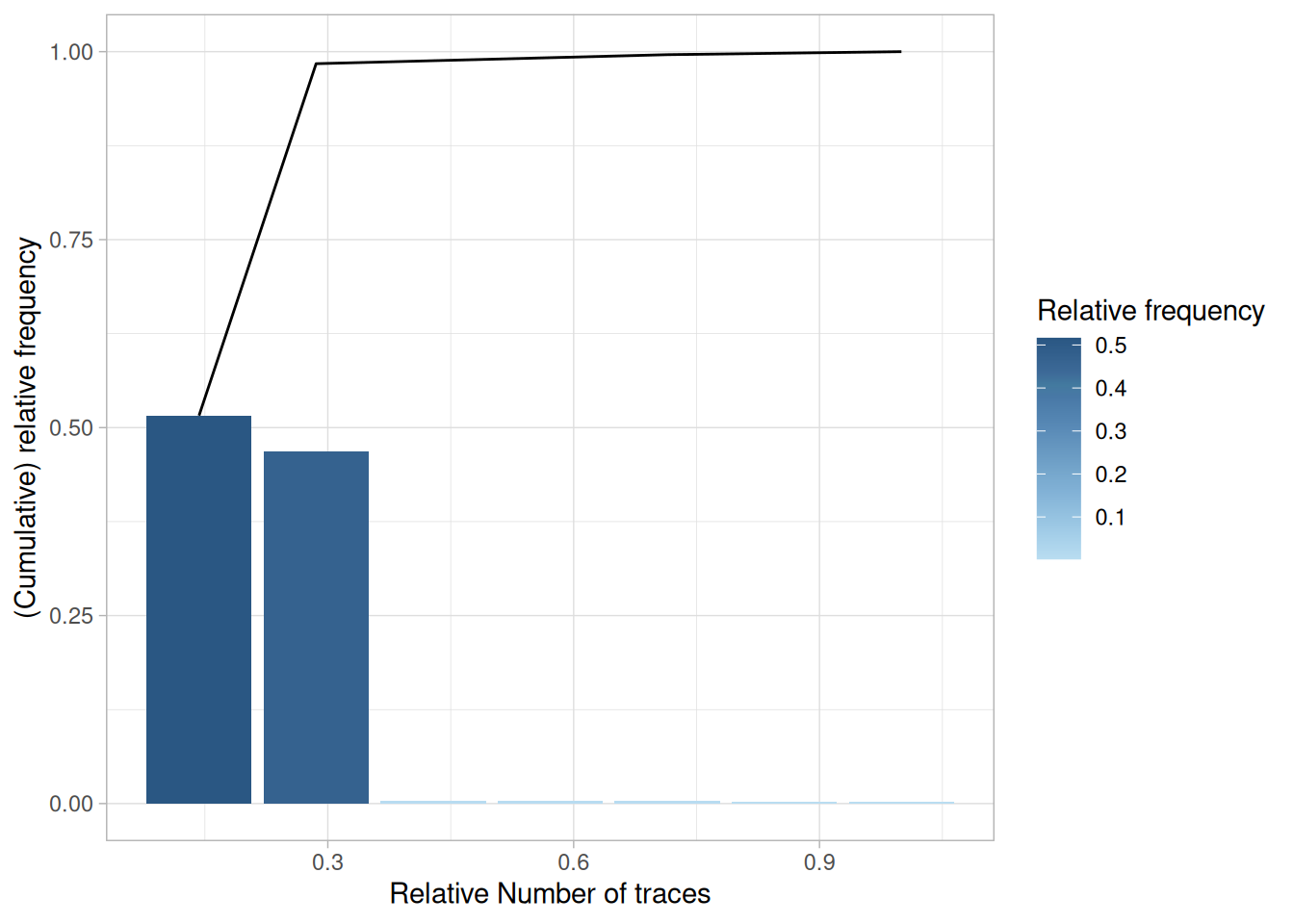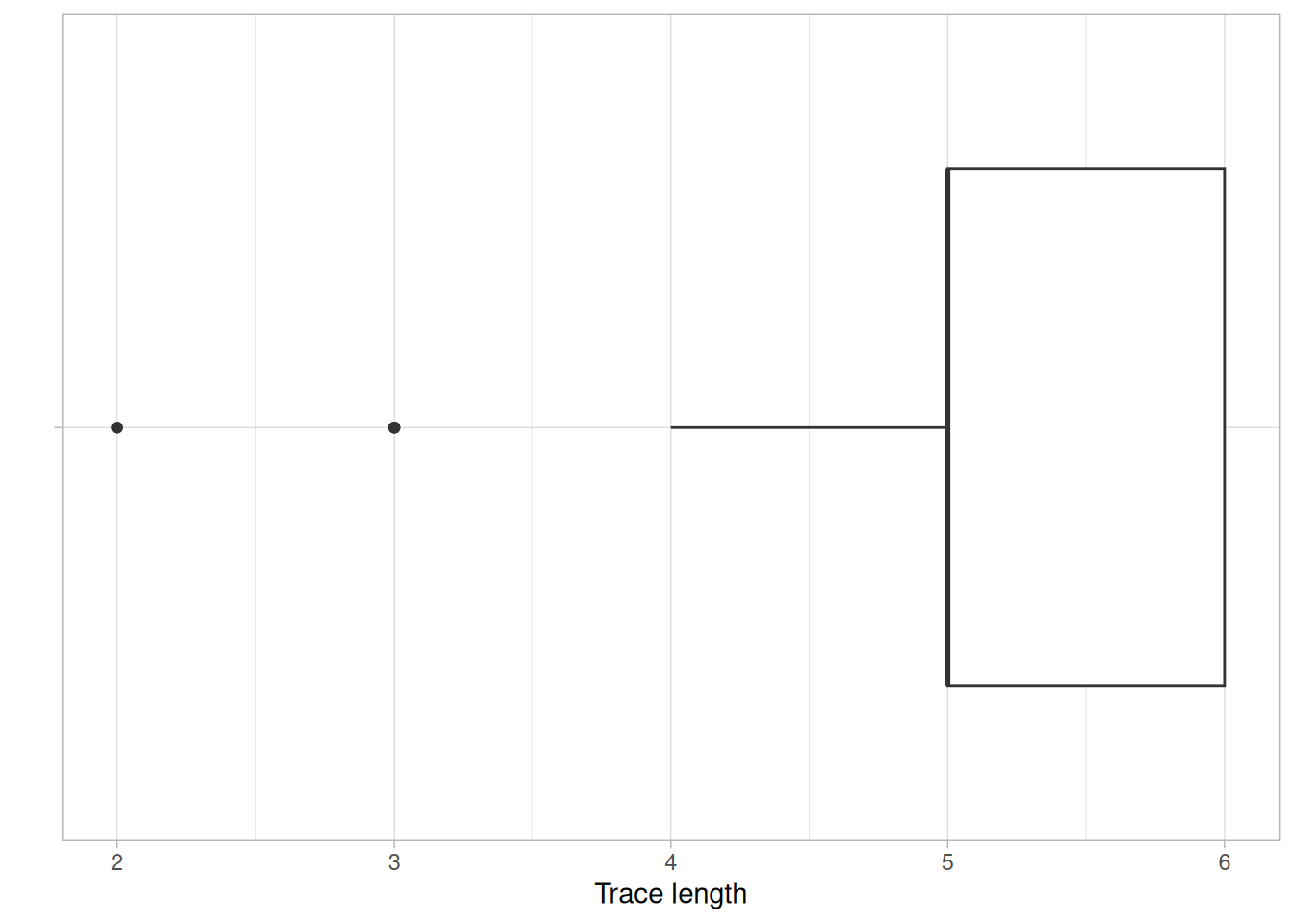# Control-flow analysis

## Metrics

### Activity Presence

Activity presence shows in what percentage of cases an activity is present. It has no level-argument.

patients %>% activity_presence() %>%
plot### Activity Frequency

The frequency of activities can be calculated using the activity_frequency function, at the levels log, trace and activity.

patients %>%
activity_frequency("activity")
## # A tibble: 7 × 3
##   handling              absolute relative
##   <fct>                    <int>    <dbl>
## 1 Registration               500   0.184
## 2 Triage and Assessment      500   0.184
## 3 Discuss Results            495   0.182
## 4 Check-out                  492   0.181
## 5 X-Ray                      261   0.0959
## 6 Blood test                 237   0.0871
## 7 MRI SCAN                   236   0.0867

### Start Activities

The start of cases can be described using the start_activities function. Available levels are activity, case, log, resource and resource activity.

patients %>%
start_activities("resource-activity")
## # A tibble: 1 × 5
##   employee handling     absolute relative cum_sum
##   <fct>    <fct>           <int>    <dbl>   <dbl>
## 1 r1       Registration      500        1       1

This shows that in this event log, all cases are started with the Registration by resource r1.

### End Activities

Conversely, the end_activities functions describes the end of cases, using the same levels: log, case, activity, resource and resource-activity.

patients %>%
end_activities("resource-activity")
## # A tibble: 5 × 5
##   employee handling              absolute relative cum_sum
##   <fct>    <fct>                    <int>    <dbl>   <dbl>
## 1 r7       Check-out                  492    0.984   0.984
## 2 r6       Discuss Results              3    0.006   0.99
## 3 r2       Triage and Assessment        2    0.004   0.994
## 4 r5       X-Ray                        2    0.004   0.998
## 5 r3       Blood test                   1    0.002   1

In contract to the start of cases, the end of cases seems to differ more frequently, although it is mostly the Check-Out activity.

### Trace Coverage

The trace coverage metric shows the relationship between the number of different activity sequences (i.e. traces) and the number of cases they cover.

patients %>%
trace_coverage("trace") %>%
plot()In the patients log, there are only 7 different traces, and 2 of them cover nearly 100% of the event log.

### Trace Length

The trace length metric describes the length of traces, i.e. the number of activity instances for each case. It can be computed at the levels case, trace and log.

patients %>%
trace_length("log") %>%
plotIt can be seen that in this simple event log, most cases have a trace length of 5 or 6, while a minority has a trace length lower than 5.

### Rework

Several metrics to measure rework (repeated work) are provided by bupaR. A distinction is made between self-loops and repetitions. A self-loop is an immediate recurrence of the same activity (i.e. no other activity in between), while a repetition is a recurrence after some other activities.

The metrics number_of_repetitions and number_of_selfloops can be used to analyse these occurrences at the levels of log, case, activity, resource and resource-activity. The metrics size_of_repetitions and size_of_selfloops (available at the same levels) provide further insight into the extent of the repeats within a single case (e.g. is it repeated only once, or multiple times?). Finally, all these metrics are able to distinguish between two types of rework: repeat rework, where the same resource does the rework, and redo rework, where the rework is done by another research. This can be specified with the type argument. Specifying type = all makes no distinction based on resources.

sepsis %>%
number_of_repetitions() 
## # A tibble: 1 × 8
##     min    q1 median  mean    q3   max st_dev   iqr
##   <dbl> <dbl>  <dbl> <dbl> <dbl> <dbl>  <dbl> <dbl>
## 1     0     0      2  1.64     3     5   1.28     3
sepsis %>%
number_of_selfloops()   
## # A tibble: 1 × 8
##     min    q1 median  mean    q3   max st_dev   iqr
##   <dbl> <dbl>  <dbl> <dbl> <dbl> <dbl>  <dbl> <dbl>
## 1     0     0      0 0.827     1    33   1.82     1
sepsis %>%
size_of_repetitions()   
## Using default type: all
## Using default level: log
## # A tibble: 1 × 8
##     min    q1 median  mean    q3   max st_dev   iqr
##   <dbl> <dbl>  <dbl> <dbl> <dbl> <dbl>  <dbl> <dbl>
## 1     1     1      2  2.67     3    58   3.72     2
sepsis %>%
size_of_selfloops() 
## Using default type: all
## Using default level: log
## # A tibble: 1 × 8
##     min    q1 median  mean    q3   max st_dev   iqr
##   <dbl> <dbl>  <dbl> <dbl> <dbl> <dbl>  <dbl> <dbl>
## 1     1     1      1  1.19     1     8  0.717     0

## Rule-based conformance

Using the packages processcheckr prodecural rules can be checked in an event log. Checking rules will add a boolean case attribute, which can be used for filtering or in analysis.

Rules can be checked using the check_rule function (see example below). It will create a new logical variable to indicate for which cases the rule holds. The name of the variable can be configured using the label argument in check_rule.

In the following example, the first rule checks the starting activity, while the second rule checks whether CRP and LacticAcid occur together.

library(bupaR)
library(processcheckR)
sepsis %>%
# check if cases starts with "ER Registration"
check_rule(starts("ER Registration"), label = "r1") %>%
# check if activities "CRP" and "LacticAcid" occur together
check_rule(and("CRP","LacticAcid"), label = "r2") %>%
group_by(r1, r2) %>%
n_cases() 
## # A tibble: 4 × 3
##   r1    r2    n_cases
##   <lgl> <lgl>   <int>
## 1 FALSE FALSE      10
## 2 FALSE TRUE       45
## 3 TRUE  FALSE     137
## 4 TRUE  TRUE      858

### Checking multiple rules

Using the function check_rules, multiple rules can be checked with one function call, by providing them as named arguments. The following code is equivalent to that above.

sepsis %>%
check_rules(
r1 = starts("ER Registration"),
r2 = and("CRP","LacticAcid")) %>%
group_by(r1, r2) %>%
n_cases() 
## # A tibble: 4 × 3
##   r1    r2    n_cases
##   <lgl> <lgl>   <int>
## 1 FALSE FALSE      10
## 2 FALSE TRUE       45
## 3 TRUE  FALSE     137
## 4 TRUE  TRUE      858

### Rule-based filtering

Instead of adding logical values for each rule, you can also immediately filter the cases which adhere to one or more rules, using the filter_rules

sepsis %>%
filter_rules(
r1 = starts("ER Registration"),
r2 = and("CRP","LacticAcid")) %>%
n_cases() 
##  858

### Rules

Currently the following declarative rules can be checked:

Cardinality rules:

• contains: activity occurs n times or more
• contains_exactly: activity occurs exactly n times
• contains_between: activity occures between min and max number of times
• absent: activity does not occur more than n - 1 times

Ordering rules:

• starts: case starts with activity
• ends: case ends with activity
• succession: if activity A happens, B should happen after. If B happens, A should have happened before.
• response: if activity A happens, B should happen after
• precedence: if activity B happens, A should have happend before
• responded_existence: if activity A happens, B should also (have) happen(ed) (i.e. before or after A)

Exclusiveness:

• and: two activities always exist together
• xor: two activities are not allowed to exist together

The available rules are explained in more detail below.

#### Cardinality rules

##### contains

Arguments:

• activity: a single activity name.
• n (default = 1): the minimum number of the times the activity should be present

Returns: cases where activity occurs n times or more.

[Example] How many cases have three or more occurences of Leucocytes?

sepsis %>%
check_rule(processcheckR::contains("Leucocytes", n = 3)) %>%
group_by(contains_Leucocytes_3) %>%
n_cases()
## # A tibble: 2 × 2
##   contains_Leucocytes_3 n_cases
##   <lgl>                   <int>
## 1 FALSE                     590
## 2 TRUE                      460
##### contains_exactly

Arguments:

• activity: a single activity name.
• n (default = 1): the exact number of the times the activity should be present

[Example] How many cases have exactly four more occurences of Leucocytes?

sepsis %>%
check_rule(contains_exactly("Leucocytes", n = 4), label = "r1") %>%
group_by(r1) %>%
n_cases()
## # A tibble: 2 × 2
##   r1    n_cases
##   <lgl>   <int>
## 1 FALSE     960
## 2 TRUE       90

Returns: cases where activity occurs n.

##### contains_between

Arguments:

• activity: a single activity name.
• min (default = 1): the minimum number of the times the activity should be present
• max (default = 1): the minimum number of the times the activity should be present

Returns: cases where activity occurs between min and max times.

[Example] How many cases have between 0 and 10 occurences of Leucocytes?

sepsis %>%
check_rule(contains_between("Leucocytes", min = 0, max = 10), label = "r1") %>%
group_by(r1) %>%
n_cases()
## # A tibble: 2 × 2
##   r1    n_cases
##   <lgl>   <int>
## 1 FALSE      38
## 2 TRUE     1012
##### absent

Arguments:

• activity: a single activity name.
• n (default = 0): the maximum number of times the activity is allowed to happen

Returns: cases where activity occurs maximum n times.

Note that absent(n = x) is equivalent to contains_between(min = 0, max = x)

[Example] How many cases have between 0 and 10 occurences of Leucocytes?

sepsis %>%
check_rule(absent("Leucocytes", n = 10), label = "r1") %>%
group_by(r1) %>%
n_cases()
## # A tibble: 2 × 2
##   r1    n_cases
##   <lgl>   <int>
## 1 FALSE    1012
## 2 TRUE       38

#### Ordering rules

##### starts

Arguments:

• activity: a single activity name

Returns: cases that start with activity.

sepsis %>%
check_rule(starts("ER Registration"), label = "r1") %>%
group_by(r1) %>%
n_cases()
## # A tibble: 2 × 2
##   r1    n_cases
##   <lgl>   <int>
## 1 FALSE      55
## 2 TRUE      995
##### ends

Arguments:

• activity: a single activity name

Returns: cases that end with activity.

[Example] How many cases end with “Release A”

sepsis %>%
check_rule(ends("Release A"), label = "r1") %>%
group_by(r1) %>%
n_cases()
## # A tibble: 2 × 2
##   r1    n_cases
##   <lgl>   <int>
## 1 FALSE     657
## 2 TRUE      393
##### succession

Arguments:

• activity_a: a single activity name
• activity_b: a single activity name

Returns: cases where (an instance of) activity_a is eventually followed by (an instance of) activity_b, if either activity_a or activity_b occurs.

[Example] How many cases is “ER Sepsis Triage” succeeded by “CRP”

sepsis %>%
check_rule(succession("ER Sepsis Triage","CRP"), label = "r1") %>%
group_by(r1) %>%
n_cases()
## # A tibble: 2 × 2
##   r1    n_cases
##   <lgl>   <int>
## 1 FALSE     229
## 2 TRUE      821
##### response

Arguments:

• activity_a: a single activity name
• activity_b: a single activity name

Returns: cases where (an instance of) activity_a is eventually followed by (an instance of) activity_b, if activity_a occurs. [Example] How many cases is “ER Sepsis Triage” followed by “CRP”, if “ER Sespis Triage” occurs.

sepsis %>%
check_rule(response("ER Sepsis Triage","CRP"), label = "r1") %>%
group_by(r1) %>%
n_cases()
## # A tibble: 2 × 2
##   r1    n_cases
##   <lgl>   <int>
## 1 FALSE     106
## 2 TRUE      944
##### precedence

Arguments:

• activity_a: a single activity name
• activity_b: a single activity name

Returns: cases where (an instance of) activity_b is preceded by (an instance of) activity_a, if activity_b occurs.

[Example] How many cases is “CRP” preceded “ER Sepsis Triage”, if “CPR” occurs.

sepsis %>%
check_rule(precedence("ER Sepsis Triage","CRP"), label = "r1") %>%
group_by(r1) %>%
n_cases()
## # A tibble: 2 × 2
##   r1    n_cases
##   <lgl>   <int>
## 1 FALSE     186
## 2 TRUE      864
##### responded_existence

Arguments:

• activity_a: a single activity name
• activity_b: a single activity name

Returns: cases where if activity_a occurs, also activity_b occurs (but not vice versa)

[Example] How many cases contain both “CRP” and “ER Sepsis Triage”, if “CPR” occurs.

sepsis %>%
check_rule(responded_existence("CRP", "ER Sepsis Triage"), label = "r1") %>%
group_by(r1) %>%
n_cases()
## # A tibble: 2 × 2
##   r1    n_cases
##   <lgl>   <int>
## 1 FALSE       1
## 2 TRUE     1049

#### Exclusiveness rules

##### and

Arguments:

• activity_a: a single activity name
• activity_b: a single activity name

Returns: cases where both activity_a and activity_b occur or both are absent

[Example] How many cases contain both “CRP” and “ER Sepsis Triage”.

sepsis %>%
check_rule(and("CRP", "ER Sepsis Triage"), label = "r1") %>%
group_by(r1) %>%
n_cases()
## # A tibble: 2 × 2
##   r1    n_cases
##   <lgl>   <int>
## 1 FALSE      44
## 2 TRUE     1006
##### xor

Arguments:

• activity_a: a single activity name
• activity_b: a single activity name

Returns: cases where either activity_a or activity_b occur, but not both.

[Example] How many cases contain “CRP” OR “ER Sepsis Triage”.

sepsis %>%
check_rule(xor("CRP", "ER Sepsis Triage"), label = "r1") %>%
group_by(r1) %>%
n_cases()
## # A tibble: 2 × 2
##   r1    n_cases
##   <lgl>   <int>
## 1 FALSE    1006
## 2 TRUE       44